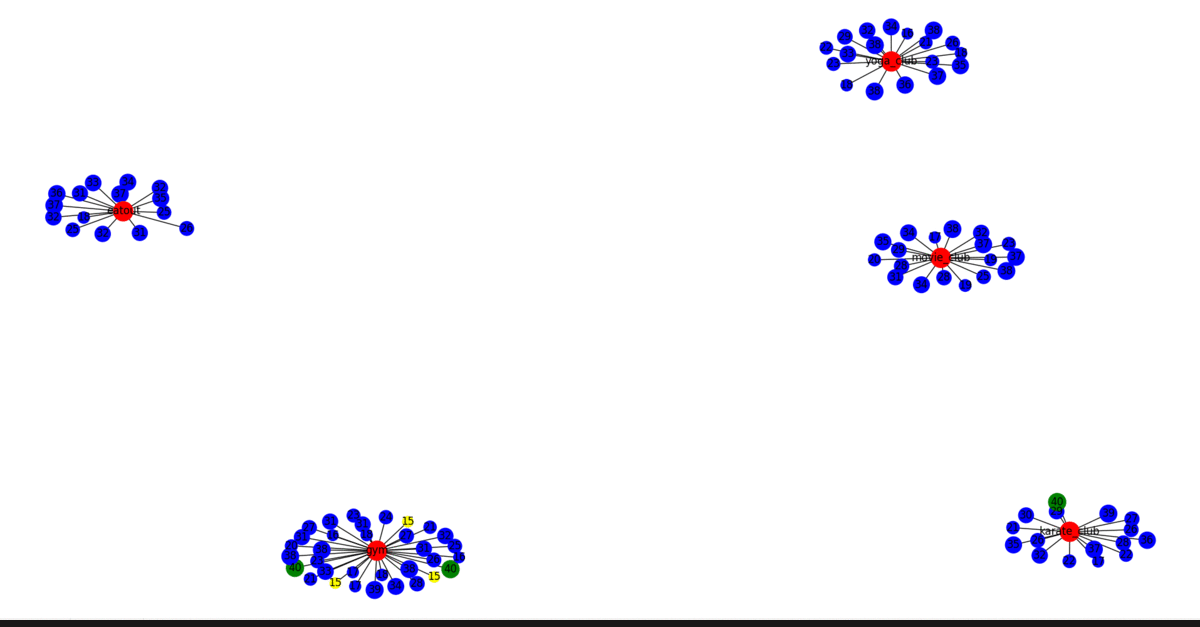Related Articles
Fatman Evolutionary Model in Social Networks
• Last Updated : 01 Oct, 2020

Prerequisite: Introduction to Social Networks

In the Fatman evolutionary model, there are 3 main concepts to make an evolutionary model i.e Homophily, Social influence, and closure.

1. Homophily- People who are similar to each other, they tend to make friends with each other.
2. Social Influence- People change their behavior and properties because of social influence.
3. Closure-  There are 3 main kinds of closures i.e triadic closure, membership closure, and foci closure.
• Triadic closure- In triadic closure, if A is a friend of B and B is a friend of C then C will eventually become friends of A.
• Membership closure- In membership closure, if A and B are in the same club then there is a tendency that they will become friends.
• Foci closure- It is the probability of becoming friends when they have the same foci.

So, According to Fatman Hypothesis, beware of fat friends, if your friend is fat then the probability of you gaining the weight becomes high.

Now the below code will show you the implementation of the above 3 concepts using the evolutionary model in python by taking an example.

Assume there is a city with a number of people, and we have everyone’s BMI. Then we will see as evolution continues people having similar BMI become friends to each other which is Homophily. Then we will create some foci like Gym etc. and see how eventually people with similar foci become a friend and then we will implement social foci.

Algorithm:

1. Create a graph with say N nodes,
2. Add edges and labels for each node.
3. Now add social foci and social foci label for each node
4. .Now implement homophily.
5. After that implement closure.
6. At last implement social influence.
7. Now Visualize the graph.

Python Code for Fatman Evolutionary Model:

## Python3

 `import` `networkx as nx``import` `matplotlib.pyplot as plt``import` `random``import` `math`` ` `# 1- Create a graph with lets say 100 nodes``# 2- Add egdes and labels.``def` `create():``    ``G ``=` `nx.Graph()``    ``G.add_nodes_from(``range``(``1``, ``101``))``    ``return` `G`` ` ` ` `def` `visualize(G):``    ``labeldict ``=` `get_labels(G)``    ``nodesize ``=` `get_size(G)``    ``color ``=` `get_colors(G)``    ``nx.draw(G, labels``=``labeldict, node_size``=``nodesize, node_color``=``color)``    ``plt.show()`` ` ` ` `def` `assign_bmi(G):``   ` `    ``for` `each ``in` `G.nodes():``        ``G.nodes[each][``'name'``] ``=` `random.randint(``15``, ``40``)``        ``G.nodes[each][``'type'``] ``=` `'person'`` ` ` ` `def` `get_labels(G):``    ``dict1 ``=` `{}``     ` `    ``for` `each ``in` `G.nodes():``        ``dict1[each] ``=` `G.nodes[each][``'name'``]``         ` `    ``return` `dict1`` ` ` ` `def` `get_size(G):``    ``array1 ``=` `[]``    ``for` `each ``in` `G.nodes():``        ``if` `(G.nodes[each][``'type'``] ``=``=` `'person'``):``            ``array1.append(G.nodes[each][``'name'``] ``*` `20``)``        ``else``:``            ``array1.append(``500``)``    ``return` `array1`` ` `# 3. Add foci nodes``def` `add_foci_nodes(G):``    ``n ``=` `G.number_of_nodes()``    ``i ``=` `n ``+` `1``    ``foci_nodes ``=` `[``'gym'``, ``'eatout'``, ``'movie_club'``, ``                  ``'karate_club'``, ``'yoga_club'``]``     ` `    ``for` `j ``in` `range``(``5``):``        ``G.add_node(i)``        ``G.nodes[i][``'name'``] ``=` `foci_nodes[j]``        ``G.nodes[i][``'type'``] ``=` `'foci'``        ``i ``+``=` `1`` ` ` ` `def` `get_colors(G):``    ``c ``=` `[]``     ` `    ``for` `i ``in` `G.nodes():``        ``if` `(G.nodes[i][``'type'``] ``=``=` `'person'``):``            ``if` `(G.nodes[i][``'name'``] ``=``=` `15``):``                ``c.append(``'yellow'``)``            ``elif` `(G.nodes[i][``'name'``] ``=``=` `40``):``                ``c.append(``'green'``)``            ``else``:``                ``c.append(``'blue'``)``        ``else``:``            ``c.append(``'red'``)``    ``return` `c`` ` ` ` `def` `get_person_nodes(G):``    ``p ``=` `[]``     ` `    ``for` `i ``in` `G.nodes():``        ``if` `(G.nodes[i][``'type'``] ``=``=` `'person'``):``            ``p.append(i)``    ``return` `p`` ` ` ` `def` `get_foci_nodes(G):``    ``f ``=` `[]``     ` `    ``for` `i ``in` `G.nodes():``        ``if` `(G.nodes[i][``'type'``] ``=``=` `'foci'``):``            ``f.append(i)``    ``return` `f`` ` ` ` `def` `add_foci_edges(G):``    ``foci_nodes ``=` `get_foci_nodes(G)``    ``person_nodes ``=` `get_person_nodes(G)``     ` `    ``for` `i ``in` `person_nodes:``        ``r ``=` `random.choice(foci_nodes)``        ``G.add_edge(i, r)`` ` `# 4. Implement Homophily``def` `homophily(G):``    ``pnodes ``=` `get_person_nodes(G)``     ` `    ``for` `u ``in` `pnodes:``        ``for` `v ``in` `pnodes:``            ``if` `(u !``=` `v):``                ``diff ``=` `abs``(G.nodes[u][``'name'``] ``-` `G.nodes[v][``'name'``])``                ``p ``=` `1` `/` `(diff ``+` `1000``)``                ``r ``=` `random.uniform(``0``, ``1``)``                ``if` `(r < p):``                    ``G.add_edge(u, v)`` ` `# 5. Implement Closure``def` `common(u, v, G):``    ``nu ``=` `set``(G.neighbors(u))``    ``nv ``=` `set``(G.neighbors(v))``    ``return` `(``len``(nu & nv))`` ` ` ` `def` `closure(G):``    ``array1 ``=` `[]``    ``for` `u ``in` `G.nodes():``        ``for` `v ``in` `G.nodes():``            ``if` `(u !``=` `v ``and` `G.nodes[u][``'type'``] ``=``=` `'person'` `or` `G.nodes[v][``'type'``] ``=``=` `'person'``):``               ` `                ``# common function will return start node, ``                ``# end node and Graph itself.``                ``k ``=` `common(u, v, G)``                ``p ``=` `1` `-` `math.``pow``(``1` `-` `0.01``, k)``                ``tmp ``=` `[]``                ``tmp.append(u)``                ``tmp.append(v)``                ``tmp.append(p)``                ``array1.append(tmp)``    ``for` `i ``in` `array1:``        ``u ``=` `i[``0``]``        ``v ``=` `i[``1``]``        ``p ``=` `i[``2``]``        ``r ``=` `random.uniform(``0``, ``1``)``        ``if` `r < p:``            ``G.add_edge(u, v)`` ` `# 6. Implement Social Influence``def` `social_influence(G):``    ``fnodes ``=` `get_foci_nodes(G)``     ` `    ``for` `i ``in` `fnodes:``        ``if` `(G.nodes[i][``'name'``] ``=``=` `'eatout'``):``            ``for` `j ``in` `G.neighbors(i):``                ``if` `(G.nodes[j][``'name'``] !``=` `40``):``                    ``G.nodes[j][``'name'``] ``=` `G.nodes[j][``'name'``] ``+` `1``                     ` `        ``if` `(G.nodes[i][``'name'``] ``=``=` `'gym'``):``            ``for` `j ``in` `G.neighbors(i):``                ``if` `(G.nodes[j][``'name'``] !``=` `15``):``                    ``G.nodes[j][``'name'``] ``=` `G.nodes[j][``'name'``] ``-` `1`` ` ` ` `G ``=` `create()``assign_bmi(G)`` ` `# to add foci field in the nodes``add_foci_nodes(G)``add_foci_edges(G)``visualize(G)`` ` `for` `t ``in` `range``(``1``, ``3``):``    ``homophily(G)``    ``visualize(G)``    ``closure(G)``    ``visualize(G)``    ``social_influence(G)``    ``visualize(G)`

Output:The output contains graphs having homophily, closure, and change in behavior i.e social influence.

Attention geek! Strengthen your foundations with the Python Programming Foundation Course and learn the basics.

To begin with, your interview preparations Enhance your Data Structures concepts with the Python DS Course.

My Personal Notes arrow_drop_up